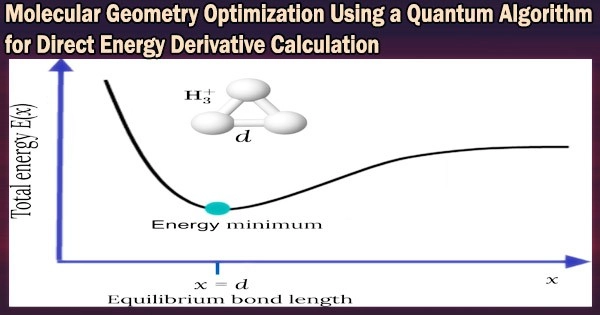Engineering

# Molecular Geometry Optimization Using a Quantum Algorithm for Direct Energy Derivative CalculationThe development of quantum computers has advanced significantly in recent years. One of the most exciting uses of quantum computers is the quantum chemistry computations for the electronic structures of atoms and molecules.

The development of geometry optimization techniques for determining the most stable structure of molecules is vital for the use of quantum chemical calculations in chemistry and related domains. Calculations of energy derivatives with regard to nuclear coordinates of molecules are necessary for the geometry optimization.

The finite difference method is one way for calculating energy derivatives. For one-dimensional systems, calculations based on this method call for at least two energy evaluations on a conventional computer.

The energy derivatives based on the finite difference approach can be calculated on a quantum computer, however, regardless of the number of degrees of freedom, with just one query. The implementation of quantum circuits necessary for performing energy derivative computations with quantum algorithms has not yet occurred.

A research group including Dr. Kenji Sugisaki, Professor Kazunobu Sato, and Professor Emeritus Takeji Takui from the Graduate School of Science at Osaka Metropolitan University has successfully extended the quantum phase difference estimation algorithm, a general quantum algorithm for the direct calculations of energy gaps, to enable the direct calculation of energy differences between two different molecular geometries.

Our latest findings bring us one step closer to applying quantum chemical calculations on a quantum computer to real-world problems. Since energy derivative calculations are used for not only molecular geometry optimizations but also various calculations for molecular properties, the application of our method is expected to play a very important role in a wide range of related fields, such as in silico drug discovery/design and materials development.

Dr. Kenji Sugisaki

This enables the estimation of energy derivatives with respect to nuclear coordinates using the finite difference approach in a single calculation.

Additionally, the study team has used the created energy derivative computations to carry out geometry optimizations of H2, LiH, BeH2, and N2 molecules without calculating the total energies, proving the value of the proposed method. The scientists also talked about how different degrees of molecular freedom can be used to create quantum circuits.

This study is the most recent in a line of articles by the researchers on quantum chemical computations.

“Our latest findings bring us one step closer to applying quantum chemical calculations on a quantum computer to real-world problems,” said Dr. Sugisaki. “Since energy derivative calculations are used for not only molecular geometry optimizations but also various calculations for molecular properties, the application of our method is expected to play a very important role in a wide range of related fields, such as in silico drug discovery/design and materials development.”